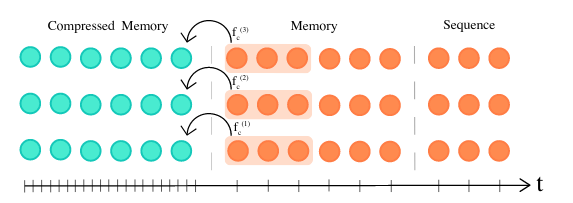# lucidrains / compressive-transformer-pytorch

Pytorch implementation of Compressive Transformers, from Deepmind

Geek Repo

Github PK Tool## Compressive Transformer in Pytorch

Pytorch implementation of Compressive Transformers, a variant of Transformer-XL with compressed memory for long-range language modelling. I will also combine this with an idea from another paper that adds gating at the residual intersection. The memory and the gating may be synergistic, and lead to further improvements in both language modeling as well as reinforcement learning.## Install

`\$ pip install compressive_transformer_pytorch`

## Usage

```import torch
from compressive_transformer_pytorch import CompressiveTransformer

model = CompressiveTransformer(
num_tokens = 20000,
emb_dim = 128,                 # embedding dimensions, embedding factorization from Albert paper
dim = 512,
depth = 12,
seq_len = 1024,
mem_len = 1024,                # memory length
cmem_len = 1024 // 4,          # compressed memory buffer length
cmem_ratio = 4,                # compressed memory ratio, 4 was recommended in paper
reconstruction_loss_weight = 1,# weight to place on compressed memory reconstruction loss
attn_dropout = 0.1,            # dropout post-attention
ff_dropout = 0.1,              # dropout in feedforward
attn_layer_dropout = 0.1,      # dropout for attention layer output
gru_gated_residual = True,     # whether to gate the residual intersection, from 'Stabilizing Transformer for RL' paper
mogrify_gru = False,           # experimental feature that adds a mogrifier for the update and residual before gating by the GRU
memory_layers = range(6, 13),  # specify which layers to use long-range memory, from 'Do Transformers Need LR Memory' paper
ff_glu = True                  # use GLU variant for feedforward
)

inputs = torch.randint(0, 256, (1, 2048))

segments = inputs.reshape(1, -1, 1024).transpose(0, 1)

logits, memories, aux_loss = model(segments, mask = masks)
logits,        _, aux_loss = model(segments, mask = masks, memories = memories)

# memories is a named tuple that contains the memory (mem) and the compressed memory (cmem)```

When training, you can use the `AutoregressiveWrapper` to have memory management across segments taken care of for you. As easy as it gets.

```import torch
from compressive_transformer_pytorch import CompressiveTransformer
from compressive_transformer_pytorch import AutoregressiveWrapper

model = CompressiveTransformer(
num_tokens = 20000,
dim = 512,
depth = 6,
seq_len = 1024,
mem_len = 1024,
cmem_len = 256,
cmem_ratio = 4,
memory_layers = [5,6]
).cuda()

model = AutoregressiveWrapper(model)

inputs = torch.randint(0, 20000, (1, 2048 + 1)).cuda()

for loss, aux_loss, _ in model(inputs, return_loss = True):
(loss + aux_loss).backward()
# optimizer step and zero grad

# ... after much training ...

# generation is also greatly simplified and automated away
# just pass in the prime, which can be 1 start token or any length
# all is taken care of for you

prime = torch.ones(1, 1).cuda()  # assume 1 is start token
sample = model.generate(prime, 4096)```